1. /
2. CBSE
3. /
4. Class 12
5. /
6. Mathematics
7. /
8. NCERT Solutions class 12...

# NCERT Solutions class 12 Maths Exercise 9.1### myCBSEguide App

Download the app to get CBSE Sample Papers 2023-24, NCERT Solutions (Revised), Most Important Questions, Previous Year Question Bank, Mock Tests, and Detailed Notes.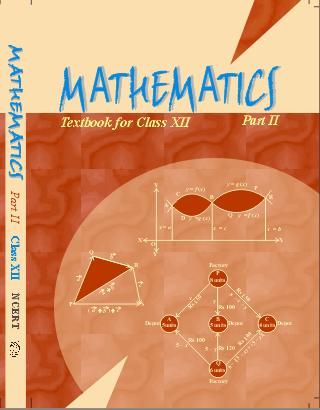## NCERT Solutions class 12 Maths Differential Equations

Determine order and degree (if defined) of differential equations given in Questions 1 to 10:

1.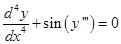Ans. Given:The highest order derivative present in the differential equation is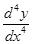and its order is 4.

The given differential equation is not a polynomial equation in derivatives as the term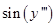is a T-function of derivative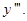Therefore the degree is not defined.

Hence, order is 4 and degree is not defined.

##### 2.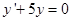Ans. Given:The highest order derivative present in the differential equation is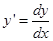and its order is 1.

The given differential equation is a polynomial equation in derivative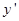and the highest power raised to highest order derivativeis one, so its degree is 1.

Hence, order is 1 and degree is 1.

##### 3.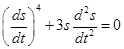Ans. Given:The highest order derivative present in the differential equation is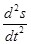and its order is 2. The given differential equation is a polynomial equation in derivatives and the highest power raised to highest order derivativeis one, so its degree is 1.

Hence, order is 2 and degree is 1.

##### 4.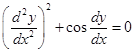Ans. Given:The highest order derivative present in the differential equation is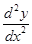and its order is 2.

The given differential equation is not a polynomial equation in derivatives as the term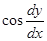is a T-function of derivative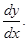Therefore the degree is not defined.

Hence, order is 2 and degree is not defined.

##### 5.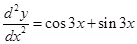Ans. Given:The highest order derivative present in the differential equation isand its order is 2.

The given differential equation is a polynomial equation in derivatives and the highest power raised to highest order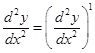is one, so its degree is 1.

Hence, order is 2 and degree is 1.

##### 6.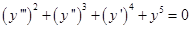Ans. Given:The highest order derivative present in the differential equation is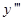and its order is 3.

The given differential equation is a polynomial equation in derivatives and the highest power raised to highest orderis two, so its degree is 2.

Hence, order is 3 and degree is 2.

7.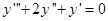Ans. Given:The highest order derivative present in the differential equation isand its order is 3.

The given differential equation is a polynomial equation in derivatives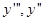andand the highest power raised to highest orderis two, so its degree is 1.

Hence, order is 3 and degree is 1.

##### 8.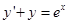Ans. Given:The highest order derivative present in the differential equation isand its order is 1.

The given differential equation is a polynomial equation in derivative. It may be noted that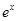is an exponential function and not a polynomial function but is not an exponential function of derivatives and the highest power raised to highest order derivativeis one so its degree is one.

Hence, order is 1 and degree is 1.

##### 9.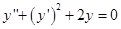Ans. Given:The highest order derivative present in the differential equation is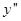and its order is 2.

The given differential equation is a polynomial equation in derivativesandand the highest power raised to highest orderis one, so its degree is 1.

Hence, order is 2 and degree is 1.

### NCERT Solutions class 12 Maths Exercise 9.1

##### 10.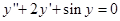Ans. Given:The highest order derivative present in the differential equation isand its order is 2.

The given differential equation is a polynomial equation in derivativeand. It may be noted that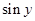is not a polynomial function of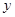, it is a T-function ofbut is not a T-function of derivatives and the highest power raised to highest order derivativeis one so its degree is one.

Hence, order is 2 and degree is 1.

### 11. The degree of the differential equation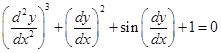is:

(A) 3

(B) 2

(C) 1

(D) Not defined

Ans. Given:……….(i)

This equation is not a polynomial in derivatives as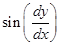is a T-function of derivativeTherefore, degree of given equation is not defined.

Hence, option (D) is correct.

### 12. The order of the differential equation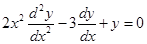is:

(A) 2

(B) 1

(C) 0

(D) Not defined

Ans. Given:The highest order derivative present in the differential equation isand its order is 2.

Therefore, option (A) is correct.

## NCERT Solutions class 12 Maths Exercise 9.1

NCERT Solutions Class 12 Maths PDF (Download) Free from myCBSEguide app and myCBSEguide website. Ncert solution class 12 Maths includes text book solutions from both part 1 and part 2. NCERT Solutions for CBSE Class 12 Maths have total 20 chapters. 12 Maths NCERT Solutions in PDF for free Download on our website. Ncert Maths class 12 solutions PDF and Maths ncert class 12 PDF solutions with latest modifications and as per the latest CBSE syllabus are only available in myCBSEguide

## CBSE App for Students

To download NCERT Solutions for class 12 Physics, Chemistry, Biology, History, Political Science, Economics, Geography, Computer Science, Home Science, Accountancy, Business Studies and Home Science; do check myCBSEguide app or website. myCBSEguide provides sample papers with solution, test papers for chapter-wise practice, NCERT solutions, NCERT Exemplar solutions, quick revision notes for ready reference, CBSE guess papers and CBSE important question papers. Sample Paper all are made available through the best app for CBSE students and myCBSEguide website.### Test Generator

Create question paper PDF and online tests with your own name & logo in minutes.### myCBSEguide

Question Bank, Mock Tests, Exam Papers, NCERT Solutions, Sample Papers, Notes